# How to Create Multiple Summary Numbers

Many customers want to display multiple card summary stats that can give them a better picture of what’s going on in the card as it helps tell the story and can highlight what the card has in it.

Description of Solution
A customized Beast Mode calculation is necessary. The “secret sauce” is the concat() function as you can add in text, and any combination of metrics inside the concat() statement. One thing to note is that this bypasses formatting options so they will need to be built into the concat() statement.

BEAST MODE LAYOUT: concat(calculation,‘ Text’, etc)

All examples below are based off of demo data.

EXAMPLES:

LOOKS LIKE: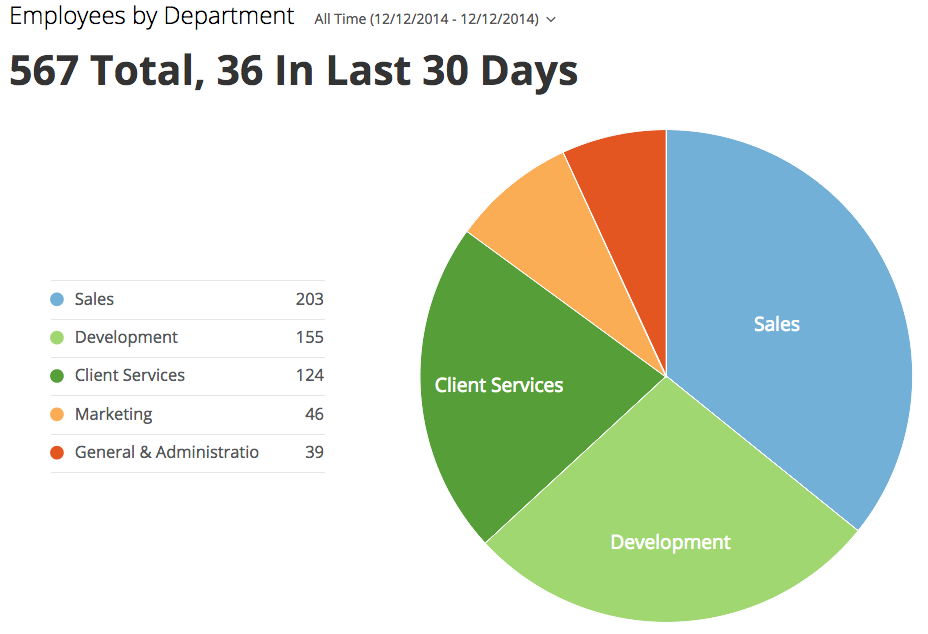BEAST MODE: concat(# calc, text, # calc, text)

concat(Sum(CASE when `Status` <> ’New' then 1 END),' Total, ',sum(case when `Date` >= date_sub(curdate(),INTERVAL 1 MONTH) then 1 else 0 end),' In Last 30 Days’)

________________________________________________________________________________

LOOKS LIKE: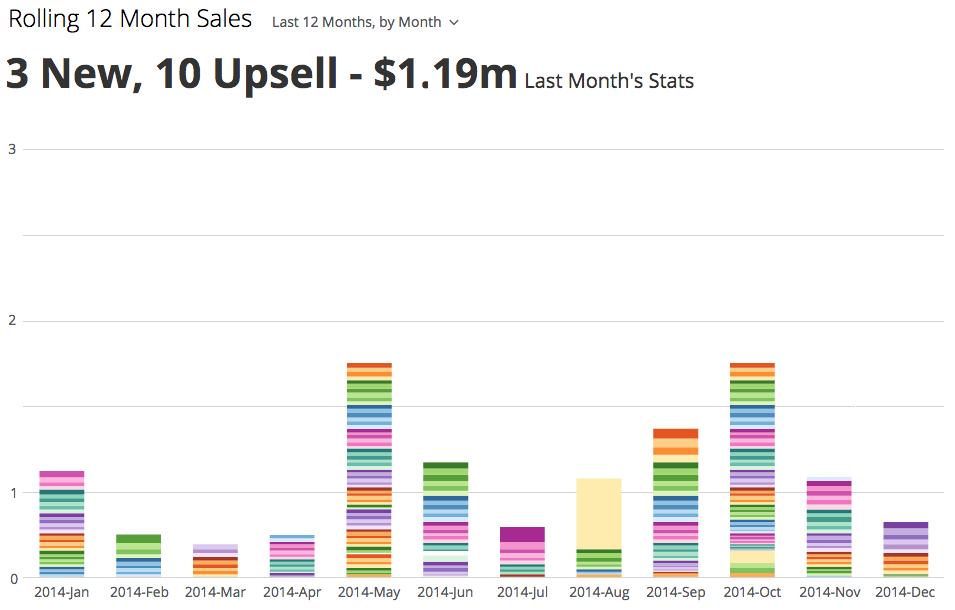BEAST MODE: concat(# calc, text, # calc, text, \$ calc, text)

concat(SUM(Case when (month(`Date`) = month(curdate()) and year(`Date`) = year(curdate()) and `Opportunity_Type__c` = 'New') then 1 else 0 end),' New, ',SUM(Case when (month(`Date`) = month(curdate()) and year(`Date`) = year(curdate()) and `Opportunity_Type__c` = 'Upsell') then 1 else 0 end),' Upsell - \$',round(SUM(Case when (month(`Date`) = month(curdate()) and year(`Date`) = year(curdate())) then `Amount` else 0 end)/1000000,2),'m’)

________________________________________________________________________________

LOOKS LIKE: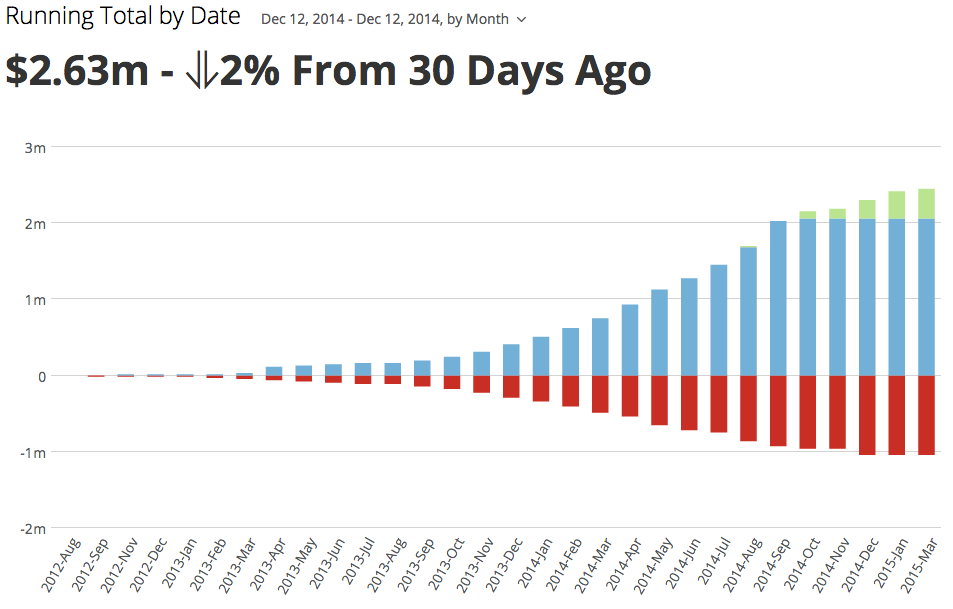BEAST MODE: concat(text, \$ calc, text, html image, # calc, text)

concat('\$',round(SUM(Case when (`Contracts__r.Date__c`<= curdate() and `Contracts__r.Status__c` = 'Accepted' and `Account.Account_Status__c` = 'Active') then `Amount` END)/1000000,2),(case when round(SUM(Case when (`Contracts__r.Date__c`<= curdate() and `Contracts__r.Status__c` = 'Accepted' and `Account.Account_Status__c` = 'Active') then `Amount` END)/SUM(Case when (`Contracts__r.Date__c`<= date_sub(curdate(), INTERVAL 1 MONTH) and `Contracts__r.Status__c` = 'Accepted' and `Account.Account_Status__c` = 'Active') then `Amount` END)-1,2) > 0 then 'm - ? ' else 'm - ? ' end),round(round(SUM(Case when (`Contracts__r.Date__c`<= curdate() and `Contracts__r.Status__c` = 'Accepted' and `Account.Account_Status__c` = 'Active') then `Amount` END)/SUM(Case when (`Contracts__r.Date__c`<= date_sub(curdate(), INTERVAL 1 MONTH) and `Contracts__r.Status__c` = 'Accepted' and `Account.Account_Status__c` = 'Active') then `Amount` END)-1,2)*100,0),'% From 30 Days Ago')

**Say “Thanks" by clicking the thumbs up in the post that helped you.
**Please mark the post that solves your problem by clicking on "Accept as Solution"

• There isn't a way to dynamically add it at the moment, but below are the two workaround solutions we have at the moment:

Solution 1:

If your results are always in the same range (ex: ###,###.00 or ##,###.00) then you could use a concat() in conjunction with a substr() function to put it in there. It may look like this: concat('\$',substr(field,1,2),',',substring(field,3,6)) to turn something like 25000.00 into \$25,000.00. The only caveat is if the format changes from ##,###.00 to ###,###.00, then it would look wrong (ex: ##,####.00).

Until we get an instr() function within beastmode where we can find the number of decimals to the left of the decimal place, then this is the best solution I've come up with if commas are required.

Solution 2:

Divide out your number to the level where commas aren't needed (ex: 74683.23 goes to 74.68k). This is done by dividing all numbers by 1000 or 1000000 depending on what your summary number range is and add either a "k" or "m" to the end.

**Say “Thanks" by clicking the thumbs up in the post that helped you.
**Please mark the post that solves your problem by clicking on "Accept as Solution"
•CoachI think you might be right... did you try saving the card so that it renders the code?  Try that with your original code that you posted

case when sum(`YTD Actual`) > 0 then

CONCAT('<div style="position:relative;"><img src="https://mitel.domo.com/page/1441105090/kpis/details/251281079" style="width:40px;height:40px;"\><span style="font-weight:bold;color:green;position:absolute;top:20px;left:44px;">',round(sum(`YTD Actual`*100),1),'%')
when sum(`YTD Actual`) < 0 then
CONCAT('<div style="position:relative;"><img src="https://mitel.domo.com/page/1441105090/kpis/details/251281079" style="width:40px;height:40px;"\><span style="font-weight:bold;color:red;position:absolute;top:15px;left:44px;">',round(sum(`YTD Actual`*100),1),'%')
end

“There is a superhero in all of us, we just need the courage to put on the cape.” -Superman
• I took several of the solutions to get it working: Scott Thompson's code with the down arrows Plus mitel's code for the color changes, plus richard's code for the dynamic jscript updates. Then it works as requested for my requirements.

«1

•ContributorTHIS IS AWESOME

•ContributorAgreed with above comment.

"When I have money, I buy books. When I have no money, I buy food." - Erasmus of Rotterdam
• I don't see any of my Beast Mode calculations in the 'Field & Function' dropdown, so I am not able to show the calculations as the Summary Number.  Is there something I need to do to enable this? Thanks

•Contributorin the Best Mode interface, make sure  you have the "Apply to Summary" check box checked for each Beast Mode field.

Domo Arigato!

**Say 'Thanks' by clicking the thumbs up in the post that helped you.
•ContributorThis is awesome Ryan. Is there possibly a way to do a line break within the summary numbers? Thanks, Nick

•ContributorDoes anybody know how to add thousands separators to numbers in CONCAT functions? I've tried using combinations of MOD and ROUND functions but haven't gotten it to work.

•ContributorHi all,

• @scherbelr Can you chime in to this conversation?

Thanks!
Dani

• There isn't a way to dynamically add it at the moment, but below are the two workaround solutions we have at the moment:

Solution 1:

If your results are always in the same range (ex: ###,###.00 or ##,###.00) then you could use a concat() in conjunction with a substr() function to put it in there. It may look like this: concat('\$',substr(field,1,2),',',substring(field,3,6)) to turn something like 25000.00 into \$25,000.00. The only caveat is if the format changes from ##,###.00 to ###,###.00, then it would look wrong (ex: ##,####.00).

Until we get an instr() function within beastmode where we can find the number of decimals to the left of the decimal place, then this is the best solution I've come up with if commas are required.

Solution 2:

Divide out your number to the level where commas aren't needed (ex: 74683.23 goes to 74.68k). This is done by dividing all numbers by 1000 or 1000000 depending on what your summary number range is and add either a "k" or "m" to the end.

**Say “Thanks" by clicking the thumbs up in the post that helped you.
**Please mark the post that solves your problem by clicking on "Accept as Solution"
•ContributorThanks @scherbelr!

One thing to note is that the SUBSTRING function only works with strings, so you have to convert a value into a string to get it to work. In my case I had a CONCAT fuction to combine the number of emails sent and the delivery rate. The original formula looked like this:

CONCAT(SUM(`NumberSent`) ,' Emails Sent, ', ROUND(SUM(`NumberDelivered`)/SUM(`NumberSent`)*100,2.0) ,'% Avg. Delivery Rate ')

and the output looked like this: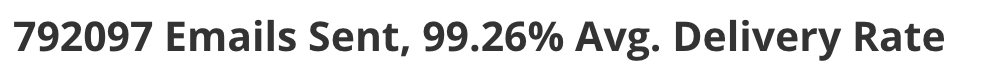I inserted the SUBSTRING function as mentioned by @scherbelr with a CONCAT function inside it. The new formula looked like this:

CONCAT((SUBSTRING((CONCAT(SUM(`NumberSent`),' Emails Sent, ')),1,3)),',',(SUBSTRING((CONCAT(SUM(`NumberSent`),' Emails Sent, ')),4,4) ),' Emails Sent, ',ROUND(SUM(`NumberDelivered`)/SUM(`NumberSent`)*100,2.0) ,'% Avg. Delivery Rate ')

and the new output looked like this: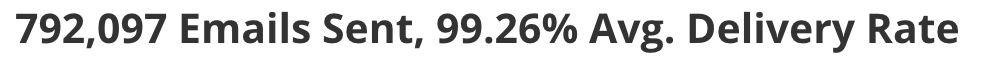• Ben,
I would try using the FORMAT function. This is not a function that is supported by Beast Mode and must be done in a dataflow. The syntax is as follows FORMAT(`column`,1) . The 1 here represents the number of places, starting from the right and going left, of where the decimal should be. If you do not have dataflow access to accomplish this you can always contact Domo Support to help you create this.
• "There is currently not a way to incorporate page breaks into the summary number. However this would make for a great enhancement request that I will be sure to file with our developers."

Thanks!
•

Has someone submitted the above as an idea? If not please do. I would suggest Ben do this so he can get credit.

Thanks!

Dani

• Try this....as a starting point. You can use the HTML color coding (or not) to your liking.

CONCAT('<p style="color: green">','\$',concat(round((sum(`JUNE_ACV`)-sum(`MAY_ACV`))/1000,0),',',round(abs(mod(sum(`JUNE_ACV`)-sum(`MAY_ACV`),1000)),0)),'</p')

BUT...when the result of the absolute value of the modulus is <100, this wont work. To remedy, you'll need to nest another case that checks to see if the result of the mod is <= 9  and then preappend a '00', then check again to see if the results of the modulus is <=99 and then preappend a '0'.

Of course this all only works if the scale of your data is < 1 million. If > 1mm, then another test and logic series is needed...

A lot of effort for a simple item.

Lastly, note that the resultant text, since it is text, will left align in a column table, which is not the standrard way to represent numbers.

Hope this helps.

• This works but I do not see where the "down arrow" symbol is configured in the calculation? When I use the same code the symbol is not displayed. It seems that I need to insert this into the calculation, yes?

Evan

• @scherbelr

Can you have a look at this one?

Thanks!

• Or maybe Domo could not be so limiting (especially for Table format Cards) and provide more summary options. This is a real hack.

• Is there now a better solution to this problem - easily adding a thousands seperator to a concatenated summary number?

• Sorry, how did you get the down arrows?

• @scherbelr

Can you provide some clarity here?

Thanks!

•CoachI believe that an alt code was used.  You can use ↑ (alt 24) or ↓ (alt 25) in your concat statement.

“There is a superhero in all of us, we just need the courage to put on the cape.” -Superman
• Oh, So there is no way to dynamically display an up or down error depending on some conditions?  As well as change colour and size of it?

• here's what I did to get this. i uploaded images to doc cards, then right-click to get image link, then concat to put up/down image based on metric trend.

-- Metric Over Arrow Version 2 for "Render Start Evolution"

case when sum(`YoY Growth`) > 0 then

CONCAT('<div style="position:relative;"><img src="https://mysite.domo.com/api/content/v1/doc-previews/510/585/PNG" style="width:40px;height:40px;"\><span style="font-weight:bold;color:green;position:absolute;top:20px;left:44px;">',round(sum(`YoY Growth`*100),1),'%')

when sum(`YoY Growth`) < 0 then

CONCAT('<div style="position:relative;"><img src="https://mysite.domo.com/api/content/v1/doc-previews/509/584/PNG" style="width:40px;height:40px;"\><span style="font-weight:bold;color:red;position:absolute;top:15px;left:44px;">',round(sum(`YoY Growth`*100),1),'%')

end

•CoachSure you can, you would just have to write it into the case statement.

case when ..... >0 then '↑' when .... <0 then '↓'

This was actually in @scherbelr 's original code:

##### concat('\$',round(SUM(Case when (`Contracts__r.Date__c`<= curdate() and `Contracts__r.Status__c` = 'Accepted' and `Account.Account_Status__c` = 'Active') then `Amount` END)/1000000,2),(case when round(SUM(Case when (`Contracts__r.Date__c`<= curdate() and `Contracts__r.Status__c` = 'Accepted' and `Account.Account_Status__c` = 'Active') then `Amount` END)/SUM(Case when (`Contracts__r.Date__c`<= date_sub(curdate(), INTERVAL 1 MONTH) and `Contracts__r.Status__c` = 'Accepted' and `Account.Account_Status__c` = 'Active') then `Amount` END)-1,2) > 0 then 'm - ? ' else 'm - ? ' end),round(round(SUM(Case when (`Contracts__r.Date__c`<= curdate() and `Contracts__r.Status__c` = 'Accepted' and `Account.Account_Status__c` = 'Active') then `Amount` END)/SUM(Case when (`Contracts__r.Date__c`<= date_sub(curdate(), INTERVAL 1 MONTH) and `Contracts__r.Status__c` = 'Accepted' and `Account.Account_Status__c` = 'Active') then `Amount` END)-1,2)*100,0),'% From 30 Days Ago')

The bolded text is where the up or down arrows would go.  I'm not sure which alt codes he used to get his arrows, but alt 24 ↑ and alt 25 ↓ should work. (instead of the ?)

I do not think that you can concat any html code in to change the color of the arrows however.

“There is a superhero in all of us, we just need the courage to put on the cape.” -Superman
• OK sorry, i'm not familiar with HTML code.

So you have a doc card for each arrow?  Green UP, and another for Red Down?

I tried this

case when sum(`YTD Actual`) > 0 then

CONCAT('<div style="position:relative;"><img src="https://mitel.domo.com/page/1441105090/kpis/details/251281079" style="width:40px;height:40px;"\><span style="font-weight:bold;color:green;position:absolute;top:20px;left:44px;">',round(sum(`YTD Actual`*100),1),'%')
when sum(`YTD Actual`) < 0 then
CONCAT('<div style="position:relative;"><img src="https://mitel.domo.com/page/1441105090/kpis/details/251281079" style="width:40px;height:40px;"\><span style="font-weight:bold;color:red;position:absolute;top:15px;left:44px;">',round(sum(`YTD Actual`*100),1),'%')
end

And here is what i got as a result

•Coach@sgraham are you wanting this in a table? or in the summary field?  I don't believe that the summary field supports html coding.  which means that you will need to use an alt code character for any symbols.  You won't be able to add graphics or web images.

I believe that @evanbench was using a table in his example.

“There is a superhero in all of us, we just need the courage to put on the cape.” -Superman
• I want a summary number card

•CoachI would try something like this then:

case when sum(`YTD Actual`) > 0 then

CONCAT('↑ ',round(sum(`YTD Actual`*100),1),'%')
when sum(`YTD Actual`) < 0 then
CONCAT('↓ ',round(sum(`YTD Actual`*100),1),'%')
end

<<I used (alt24) for the up arrow and (alt 25) for the down>>

You could also use alt 30 ▲ and alt 31 ▼

“There is a superhero in all of us, we just need the courage to put on the cape.” -Superman
• OK, that works, but how do i get color?  I've seen many posts where people are saying you can insert HTML for colour, but i can't seem to get that to work

•CoachI think you might be right... did you try saving the card so that it renders the code?  Try that with your original code that you posted

case when sum(`YTD Actual`) > 0 then

CONCAT('<div style="position:relative;"><img src="https://mitel.domo.com/page/1441105090/kpis/details/251281079" style="width:40px;height:40px;"\><span style="font-weight:bold;color:green;position:absolute;top:20px;left:44px;">',round(sum(`YTD Actual`*100),1),'%')
when sum(`YTD Actual`) < 0 then
CONCAT('<div style="position:relative;"><img src="https://mitel.domo.com/page/1441105090/kpis/details/251281079" style="width:40px;height:40px;"\><span style="font-weight:bold;color:red;position:absolute;top:15px;left:44px;">',round(sum(`YTD Actual`*100),1),'%')
end

“There is a superhero in all of us, we just need the courage to put on the cape.” -Superman